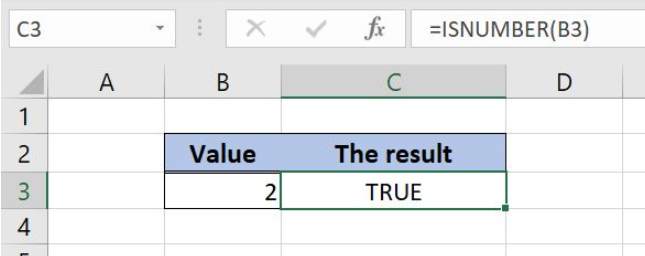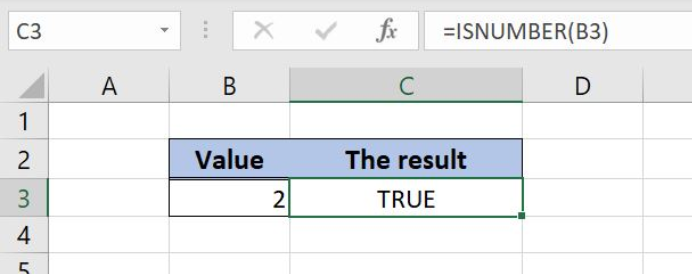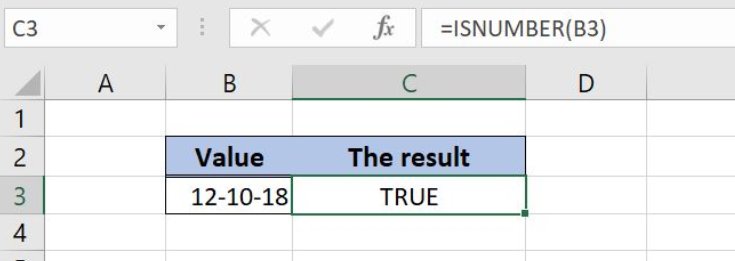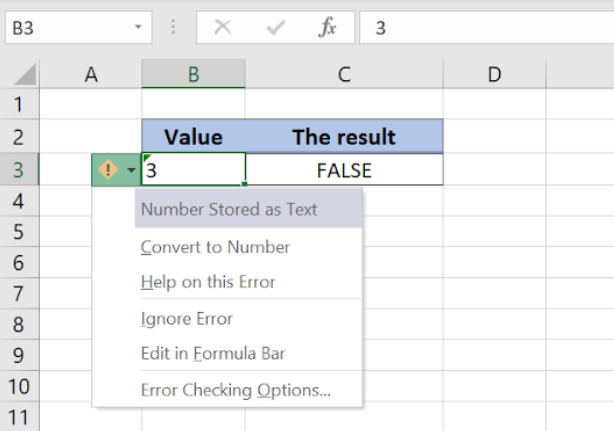Get instant live expert help with Excel or Google Sheets“My Excelchat expert helped me in less than 20 minutes, saving me what would have been 5 hours of work!”

#### Post your problem and you’ll get Expert help in seconds.

Your message must be at least 40 characters
Our professional Expert are available now. Your privacy is guaranteed.

# Excel ISNUMBER Function

Excel allows a user to check if a value is numeric, by using the ISNUMBER function. This step by step tutorial will assist all levels of Excel users in checking if a value or a value of a cell isnumeric.Figure 1. The result of the ISNUMBER function

## Syntax of the ISNUMBER Formula

The generic formula for the ISNUMBER function is:

`=ISNUMBER(value)`

The parameter of the ISNUMBER function is:

• value – a value or a cell which we want to check if it is numeric.

If a selected value is numeric, the function will return TRUE as a result. Otherwise, it will return FALSE.

## Check If Cells Value is Numeric by the ISNUMBER function

In our example, we want to check if the value from the cell B3 is a number. In B3, we put 2 as the value.

The formula is:

`=ISNUMBER(B3)`

The parameter value is the cell B3, while the result is in the cell C3.

To apply the ISNUMBER function, we need to follow these steps:

• Select cell C3 and click on it
• Insert the formula: `=ISNUMBER(B3)`
• Press enterFigure 2. Using the ISNUMBER function to check if the value in the cell is numeric

The value in B3 is numeric, so the function returns Boolean TRUE as a result in the cell C3.

## Notes

There are two specific cases when using the ISNUMBER function:

• Checking if date value is numeric. The function will always return TRUE for the date because Excel stores dates as numbers:Figure 3. The ISNUMBER checking a date

The date 12-10-18 is internally stored as 43385 is Excel. Because of that, the ISNUMBER returns TRUE as a result.

• Checking numbers stored as text. If the number is stored as a text, the ISNUMBER will return FALSE:Figure 4. The ISNUMBER checking the number stored as text

In the cell B3, we put 3, but the cell is formatted as text. Because of that, the ISNUMBER returns FALSE as a result.

Most of the time, the problem you will need to solve will be more complex than a simple application of a formula or function. If you want to save hours of research and frustration, try our live Excelchat service! Our Excel Experts are available 24/7 to answer any Excel question you may have. We guarantee a connection within 30 seconds and a customized solution within 20 minutes.

### Did this post not answer your question? Get a solution from connecting with the expert.Another blog reader asked this question today on Excelchat:## Subscribe to Excelchat.coAnother blog reader asked this question today on Excelchat: